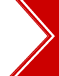Ascent's Courses Business Partnership Jobs @ Ascent Testimonials Success Stories

Analytical Geometry - Quant/Math - CAT 2013

Question 4 the day: April 11, 2003

The question for the day is from the topic of Analytical Geometry.
Find the equation of a line whose intercepts are twice of the line 3x  2y  12 = 0

 (1) 3x  2y = 24 (2) 2x  3y = 12 (3) 2x  3y = 24 (4) None of these

Solution:

Put x = 0, then y = - 6

Therefore, y intercept is  6

Put y = 0, then x = 4

Therefore, x intercept is 4

Hence the intercepts of the required line are 8 and  12

Hence the equation of the line is (x/8) + (y/-12) = 1

-12x + 8y = -96

3x  2y = 24.

 Home | Question Bank | Top B-Schools | US B-Schools | GD-PI | Forums | GRE Preparation Chennai | Doubt Fire © 2002 - 10 ASCENT Education all rights reserved.